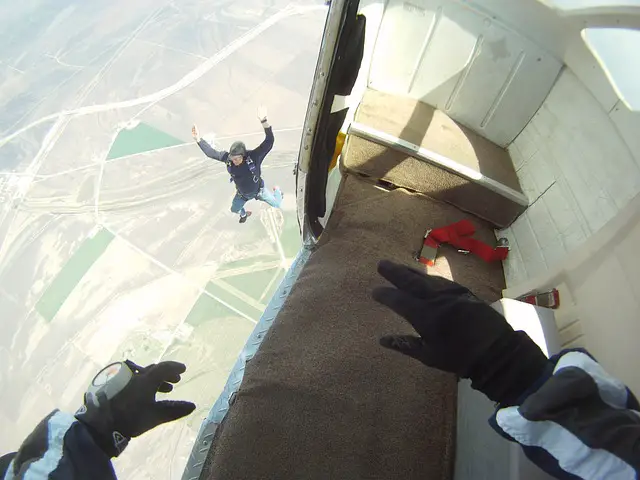# PHYSICS TEACHER

## Physics Fundamentals BLOG for K12RSS Feed

# gravity### What will happen to a ball thrown vertically upwards?

##### Gravitation Motion

Motion of a ball thrown vertically upwards You must have seen someone throwing a ball vertically upwards or probably you have thrown it yourself. Yes, the ball goes up for a while and then stops and starts to come down and touches the ground with a bang if you can’t catch it. Let’s discuss the phases### Free fall, Acceleration due to Gravity and Kinetic Energy

##### Force Gravitation Motion Work, Energy, Power

Weight of any object is a force applied on the object by earth’s gravitational pull towards the center of the earth. We know the equation of a force = mass * acceleration. i.e.      F=m*a…….(1) When we talk of weight force then the equation becomes like this: Weight(W)= mass * acceleration due to gravity